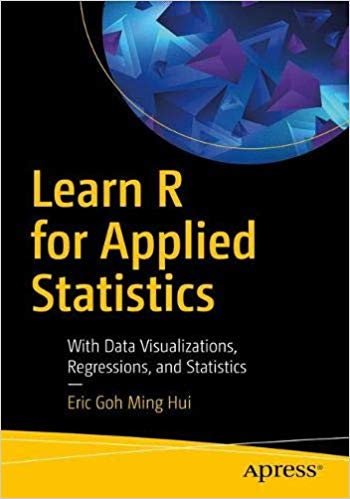# Learn R for Applied Statistics – pdf – 电子书免费下载Gain the R programming language fundamentals for doing the applied statistics useful for data exploration and analysis in data science and data mining. This book covers topics ranging from R syntax basics, descriptive statistics, and data visualizations to inferential statistics and regressions. After learning R’s syntax, you will work through data visualizations such as histograms and boxplot charting, descriptive statistics, and inferential statistics such as t-test, chi-square test, ANOVA, non-parametric test, and linear regressions.

Learn R for Applied Statistics is a timely skills-migration book that equips you with the R programming fundamentals and introduces you to applied statistics for data explorations.

What You Will Learn

• Discover R, statistics, data science, data mining, and big data
• Master the fundamentals of R programming, including variables and arithmetic, vectors, lists, data frames, conditional statements, loops, and functions
• Work with descriptive statistics
• Create data visualizations, including bar charts, line charts, scatter plots, boxplots, histograms, and scatterplots
• Use inferential statistics including t-tests, chi-square tests, ANOVA, non-parametric tests, linear regressions, and multiple linear regressions
Who This Book Is For

Those who are interested in data science, in particular data exploration using applied statistics, and the use of R programming for data visualizations.

ISBN:1484241991

## 锦瑟无端五十弦，一弦一柱思华年

### 觉得文章有用就打赏一下文章作者

#### 支付宝扫一扫打赏#### 微信扫一扫打赏